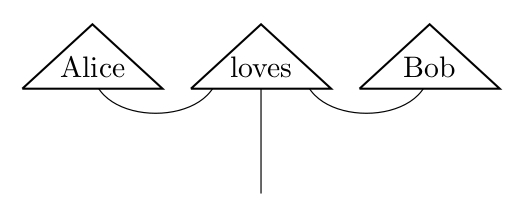# discokitty ![Version](https://img.shields.io/badge/version-0.1.0-blue.svg) An educational implementation of the DisCoCat framework as described in *"Mathematical Foundations for a Compositional Distributional Model of Meaning"* ([link](https://arxiv.org/abs/1003.4394)) by Coecke, Sadrzadeh and Clark.*[Sunglasses Kitty](https://thenounproject.com/Gilleas/collection/kitty-emoticons/?i=359412#) by Rikki Lorie, licensed under Creative Commons* ## Usage example Please note that this library is work in progress and it is possible that substantial changes will be made. In the following example we work in the category of relations declaring the meaning and grammar type of some words and then we evaluate an example sentence. ``` haskell module Discokitty.Examples.AliceAndBob where import Discokitty import Discokitty.Models.Diagrams import Discokitty.Models.Rel -- We first declare an universe with all the possible basis words, -- both nouns and sentences. The rest of the types are parameterized -- by this universe. data Universe = Alice | Bob | IsTrue | IsFalse deriving (Eq, Ord, Show) -- We choose to use the category of relations for this example, and we -- declare a term to be a word in the category of relations for our -- given universe. type Term = Words (Rel Universe) -- We give meaning to some terms. Relations are described as subsets using -- "relation", and the Lambek grammatical type must be written at the end. alice :: Term alice = Words { meaning = relation [ [ Alice ] ] , grammar = [N] , text = "Alice" } bob :: Term bob = Words { meaning = relation [ [ Bob ] ] , grammar = [N] , text = "Bob" } loves :: Term loves = Words { meaning = relation [ [ Alice , IsTrue , Bob ] ] , grammar = [ L N , S , R N ] , text = "loves" } -- In our example sentence, we evaluate "Alice loves Bob". -- This produces the following output: -- > [[IsTrue]] of grammar type [S] example :: [Term] example = sentence [alice , loves , bob] @@@ [S] -- We can also generate Tikz diagrams. exampleDiagram :: String exampleDiagram = tikzDiagrams [alice , loves , bob] ``` The generated tikz diagram looks as follows.